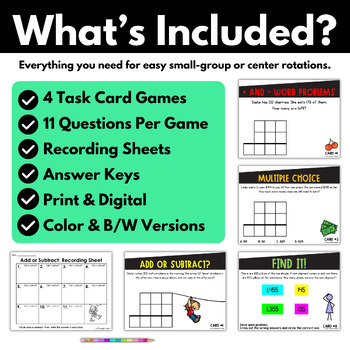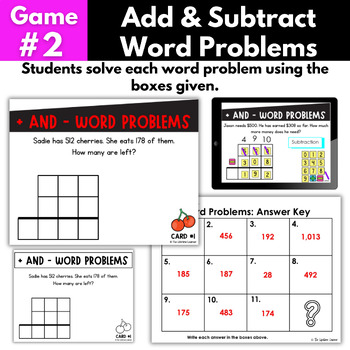Rated 4.85 out of 5, based on 20 reviews
20 Ratings;
3rd
Subjects
Resource Type
Standards
Formats Included
• Zip
•Google Apps™
Pages
40 pages
Report this resource to TPTThe Teacher-Author indicated this resource includes assets from Google Workspace (e.g. docs, slides, etc.).

#### What educators are saying

I loved these task cards. They helped the students so much so they could line everything up and then work the problem. Thank you.
Love this activity. Its hard to find digital activities to work on regrouping and problem solving. The one group I had was not quite ready to subtract with double zeros, but we managed through it.
##### Also included in
1. This is the ULTIMATE third grade math bundle for teaching online and in person!! Do your 3rd grade students need more practice with third grade math standards? Are you looking for engaging, rigorous games that will help students with these important skills (without taking hours to prep!)? Look no fu
Price \$102.00Original Price \$204.00Save \$102.00

### Description

Do your third grade students need more practice with addition and subtraction word problems within 1000? Are you looking for engaging, rigorous games that will help students with this important skill (without taking hours to prep!)? Look no further! These print AND digital activities will meet your grade 3 students' needs. There are four addition and subtraction word problem games to help students practice their math skills - in a fun way! They align with 3.NBT.2. These 3rd grade math activities are great for tackling adding and subtracting 3 digit numbers.

WHAT'S INCLUDED? (PRINT AND DIGITAL)

Game 1: Decide the Operation

Students practice solving multi-digit addition and subtraction word problems with regrouping virtually. Students decide if each problem is addition or subtraction. They drag over the correct operation and then solve the problem too! There are 3 versions included: dragging the numbers version, numbers already filled in for students version, and typing the numbers version.

Game 2: Circle the Keywords

Students practice solving addition and subtraction word problems with regrouping during distance learning. Students do this by circling the keyword in red and then solving each equation. There are 3 versions included: dragging the numbers version, numbers already filled in for students version, and typing the numbers version.

Game 3: Multiple Choice

Students solve each math equation on the page and then circle the correct answer in this multiple choice game. There are 3 versions included: dragging the numbers version, numbers already filled in for students version, and typing the numbers version.

Game 4: Delete It

Students solve each math problem on the page and then delete the answers that are wrong. There are 4 answers on each slide so students will have to figure out the 3 wrong answers and delete them so only the correct answer remains.

HOW TO IMPLEMENT:

There are 11 problems included in each of the 4 games (total of 44 questions).

Digitally: The first problem is always an example for students so they know what to do. I would recommend completing 5 questions during your lesson together and assigning the other 5 for students to complete independently.

Print: Print the task cards out and put them in a math center. Have students record answers on the recording sheet provided. Students can use the self-checking answer key to check their work. You can choose between the color and black and white option.

-fun and engaging

-aligned to common core

-no prep

-2 versions: print and digital / online / remote / distance learning

-rigorous

-color and black and white

-easy to use format

These third-grade math worksheet alternatives are perfect as math assessments, quick checks, quizzes, homework, remediation materials, extra practice, work to do at home, pre-assessments, post-assessments, unit tests, and more.

✅4 games

✅4 games

✅practices common core standard 3.NBT.2

✅multiple versions for different learning styles

✅3rd grade Common Core and TEKS aligned

WHAT DO YOU NEED TO ACCESS DIGITALLY?

-This digital resource was created with Google Slides™.

WAYS TO USE:

-partners

-small groups

-math centers

-distance learning

-enrichment

-remediation

-guided math

-independent practice

-spiral review

-----------------------------------------------------------------------------------------------------------------

Aligns with Common Core 3.NBT.2:

• Fluently add and subtract within 1000 using strategies and algorithms based on place value, properties of operations, and/or the relationship between addition and subtraction.

This aligns with 3rd Grade Texas TEKS:

• TEKS 3.4A: solve with fluency one-step and two-step problems involving addition and subtraction within 1,000 using strategies based on place value, properties of operations, and the relationship between addition and subtraction
• TEKS 3.5A: represent one- and two-step problems involving addition and subtraction of whole numbers to 1,000 using pictorial models, number lines, and equations

This aligns with Florida BEST Standards (B.E.S.T.)

• MA.3.NSO.2.1 Add and subtract multi-digit whole numbers including using a standard algorithm with procedural fluency.
• MA.3.AR.1.2 Solve one- and two-step real-world problems involving any of four operations with whole numbers.

-----------------------------------------------------------------------------------------------------------------

Round to the Nearest 10 within 100

Round to the Nearest 10 within 1000

Round to the Nearest 100

Multi-Digit Subtraction

Multiply by Tens

Multiplication Using Arrays

Multiplication Using Equal Groups

Multiplication Using Skip Counting

Multiplication Using All 4 Strategies

Division Using Equal Groups

Division Using Repeated Subtraction

Division Using Skip Counting

Division Games Using Any Strategy

Division Using All 4 Strategies

Multiplication and Division Word Problems

Distributive Property

Multiplication and Division Fact Families

Multiplication and Division Missing Factors

Multiplication Fact Fluency Games Bundle

Multi-Step Word Problems

Patterns

Identify Fractions

Fractions on Number Lines

Equivalent Fractions

Comparing Fractions

Fractions as Whole Numbers

Telling Time

Elapsed Time

Bar Graphs and Pictographs

Capacity and Mass

Measure with Rulers

Finding Area

Finding Perimeter

Area and Perimeter Word Problems

Area and Perimeter Missing Sides

Rectilinear Area

Geometry

3rd Grade Math Games BUNDLE (Save 50%)

Want a Room Transformation for this Skill?

Bowling Room Transformation

Space Room Transformation

Weather Room Transformation

----------------------------------------------------------------------------------

Other Information:

Follow me and be notified when new products are uploaded. New products are always 50% off for the first 24 hours they are posted.

Thank you so much,

Purchasing this product grants permission for use by one teacher in his or her own classroom. If you intend to share with others, please purchase an additional license at a discount!

Questions?

Total Pages
40 pages
Included
Teaching Duration
2 Weeks
Report this resource to TPT
Reported resources will be reviewed by our team. Report this resource to let us know if this resource violates TPT’s content guidelines.

### Standards

to see state-specific standards (only available in the US).
Fluently add and subtract within 1000 using strategies and algorithms based on place value, properties of operations, and/or the relationship between addition and subtraction.